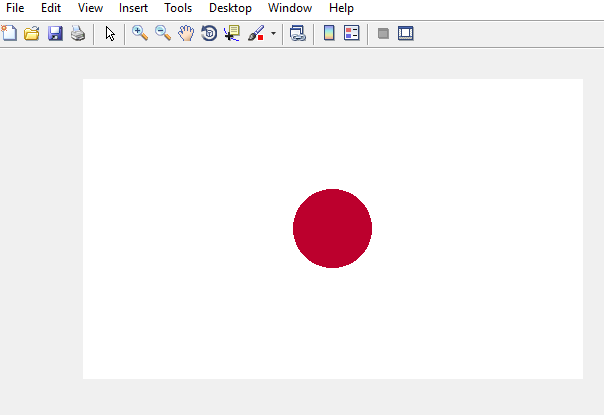Related Articles
How to draw Japan flag using MATLAB
• Last Updated : 17 Feb, 2019

Prerequisite: RGB Image Representation

MATLAB, also called Matrix Laboratory, is a numerical computing environment and a platform for programming languages. It was designed and developed by MathWorks. MATLAB is a framework that allows us to perform matrix manipulations, implementing algorithms, plotting functions and data, creating user-interfaces and interfacing with programs that are written in different programming languages i.e. C, C++, python, java etc..

How to draw a colored image in MATLAB ?
A colored image can be represented as a 3 order matrix. The first order is for the rows, the second order is for the columns and the third order is for specifying the color of the corresponding pixel. Here we use the RGB color format, so the third order will take 3 values of Red, Green and Blue respectively. The values of the rows and columns depend on the size of the image.

Approach to draw the flag of japan:

• Make a zero matrix of 3 order that has dimensions (300, 500, 3) where 300 denotes the number of pixels for rows, 600 denotes the number of rows and 3 denotes the color coding in RGB format.
• Color the whole matrix in white color first. RGB for red color is (255, 255, 255).
`I(:, :, :)=255;`
• Apply loop on rows and columns and implement the equation of the circle such that we get a circle in the center of the flag and color it crimson glory using RGB format.
Equation of circle:

`((x-h)^2 - (y-k)^2)=r^2`

where (h, k) are the centers, (x, y) are co-ordinates of x-axis and y-axis and is the radius of the circle.
rgb format for crimson glory color is (188, 0, 45).

Below is the code:

 `% create a 2-D matrix and paint it white``I = uint8(zeros(300, 500, 3))+255; `` ` `%the center point 1 through which the circle will pass``circle_center1=150; `` ` `%the center point 2 through which the circle will pass``circle_center2=250;  `` ` `radius=6.32;    ``% radius of the circle``x=i;            ``% x-axis co-ordinate``y=j;            ``% y-axis co-ordinate`` ` `%loop for rows i.e. for x-axis`` ``for` `i = 101:200``  ` `     ``%loop for columns i.e. for y-axis``      ``for` `j = 101:300``       ` `         ``%applying the equation of circle to make the circle in the center.``          ``if` `round(sqrt((i-circle_center1)^2 + (j-circle_center2)^2)) < radius^2``           ` `            ``% fill the circle with crimson glory``            ``% color using RGB color representation.``            ``I(i, j, 1) = 188;``            ``I(i, j, 2) = 0;``            ``I(i, j, 3) = 45;``             ` `          ``end`        `          ` `      ``end`  `% end column loop.`` ``end`       `% end row loop.``  ` `% show the image formed. ``figure, imshow(I);  `

Output:Attention geek! Strengthen your foundations with the Python Programming Foundation Course and learn the basics.

To begin with, your interview preparations Enhance your Data Structures concepts with the Python DS Course. And to begin with your Machine Learning Journey, join the Machine Learning – Basic Level Course

My Personal Notes arrow_drop_up